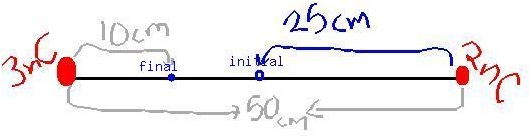Question# Two stationary point charges +3 nC and + 2nC are separated bya distance of 50cm

Other
ANSWEREDTwo stationary point charges $$+3 nC$$ and $$+ 2nC$$ are separated bya distance of 50cm. An electron is released from rest at a pointmidway between the two charges and moves along the line connectingthe two charges. What is the speed of the electron when it is 10cm from $$+3nC$$ charge?
Besides the hints I'd like to ask you to give me numericalsolution so I can verify my answer later on. It would be nice ifyou could write it out, but a numerical anser would be fine alongwith the hint how to get there.2021-03-31From the diagram we can notice that the motion is along x-axis.
$$\displaystyle{x}_{{i}}={25}{c}{m},{x}_{{f}}={10}{c}{m}$$
Consider $$\displaystyle{x}_{{i}}={0}$$ and the positve x-axis toward the 3nC charge, so $$\displaystyle{x}_{{f}}={15}{c}{m}$$.
$$\displaystyle{x}_{{f}}-{x}_{{i}}={15}{c}{m}$$
F at this point equal to
$$\displaystyle\sum{F}={F}_{{{b}{y}{3}{n}{C}}}+{F}_{{{b}{y}{2}{n}{C}}}$$
$$\displaystyle{F}_{{{b}{y}{3}{n}{C}}}={k}{\frac{{{3}\times{10}^{{-{6}}}-{1.6}\times{10}^{{-{19}}}}}{{{10}}}}\times{10}^{{-{2}}}$$
$$\displaystyle{F}_{{{b}{y}{2}{n}{C}}}={k}{\left({2}\times{10}^{{-{6}}}-{1.6}\times{10}^{{-{19}}}\right\rbrace}{\left\lbrace{40}\right\rbrace}\times{10}^{{-{2}}}$$
Caculate both forces and then ,
$$\displaystyle{a}{x}={\frac{{\sum{F}}}{{{m}_{{{e}le{c}{t}{r}{o}{n}}}}}}$$
when u get the acceleration u can get the velocity usingconstant acceleration motion equations ,
Use :
$$\displaystyle{v}_{{x}}=\sqrt{{{2}{a}{x}{\left({x}{f}-\xi\right)}}}$$
u know that the initial velocity is zero as long we assumedthe motion started from the origin
and $$(xf-xi) = 15cm$$